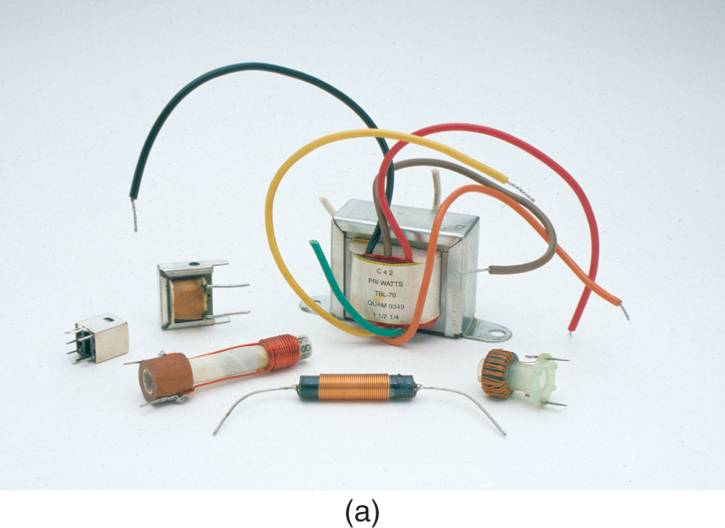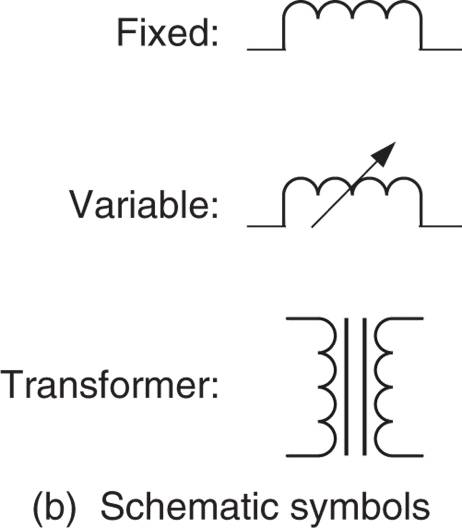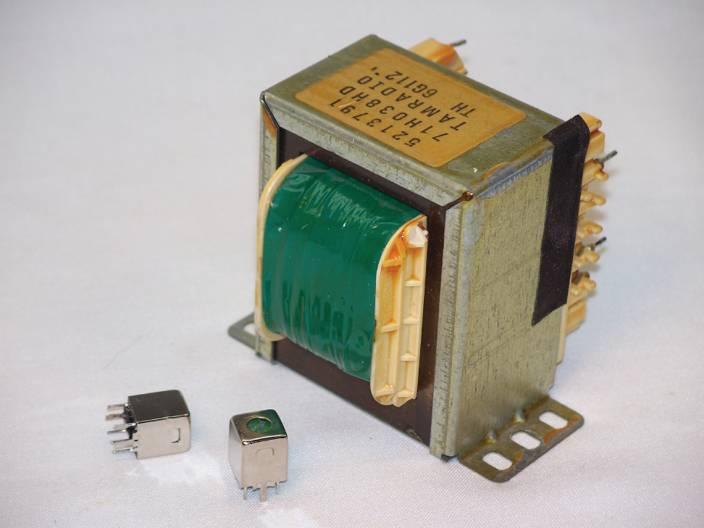Home / Electrical Circuits / Electrical Components and Symbols

# Electrical Components and Symbols

Want create site? Find Free WordPress Themes and plugins.

Electrical and electronic systems contain circuits. Loosely defined, a Circuit is a group of components that performs a specific function. The function performed by a given circuit depends on the components used and how they are connected together. Nearly all circuits, no matter how complex, are made up of the same basic types of components.

While the number of components used and their arrangement varies from one circuit to another, the types of components used are almost universal. In this article, we will take a brief look at the basic types of components, the symbols used to represent them in electrical diagrams, and the units that are used to express their values.

Circuit: A group of components that performs a specific function.

Resistors

We have all heard the term current as it applies to a river or stream: it is the flow of water from one point to another. In electrical circuits, the term current is used to describe the flow of electricity from one point in a circuit to another. A Resistor is a component that is used to restrict the current in a circuit, just as a valve can be used to restrict the flow of water through a pipe. Some resistors are shown in Figure 1a.Figure 1a Resistors

The resistor symbols shown in Figure 1b are used in Schematic Diagrams, which show you how the components in a circuit are interconnected. The schematic symbol for a resistor indicates whether its value is fixed or variable.

Resistors: A component that is used to restrict (limit) current.Figure 1b Resistors Symbols: Variable and Fixed Resistor Symbols

Capacitors

A Capacitor is a component that stores energy in an electric field. Capacitors are used in a wide variety of electric and electronic circuits. Several-capacitors and the common capacitor schematic symbols are shown in Figure 2.Figure 2: Capacitors and their Symbols: Variable and Fixed Capacitors Symbols

Capacitors: A component that stores energy in an electric field, also called a condenser.

Inductors

An Inductor is a component that stores energy in a magnetic field. Though both inductors and capacitors store energy, they are very different in terms of their construction and characteristics. Several types of inductors and the basic inductor schematic symbols are shown in Figure 3. Inductors are often referred as Coils or Chokes.Figure 3: (a) & (b): Inductors: Variable and Fixed Inductors symbols

Inductors: A component that stores energy in a magnetic field, also called a coil or a choke.

Transformers

A Transformer is a component that contains one or more inductors that are wrapped around a single physical structure. Transformers are used to:

• Change one voltage level to another.
• Transfer electrical energy from one circuit to another.

Transformers come in all types and sizes and are used in most electrical and electronic systems. A Step-Down Transformer decreases its input voltage to a lower level. A Step-Up Transformer increases its input voltage to a higher level. Some smaller transformers are shown in Figure 4.Figure 4 Transformers.

Switches

A Switch is a component that is used to make or break an electrical connection. Several common types of switches are shown in Figure 5.Figure 5 Switches

Fuses and Circuit Breakers

A Fuse is a component that automatically breaks an electrical connection. If the current increases beyond a certain value, if some condition, like an electrical short causes the current in a circuit to suddenly increase, the circuit fuse “burns open” to break the current path and protect the circuit. Some typical fuses are shown in Figure 6.Figure 6 Fuses and circuit breakers

A fuse can only protect a circuit once. If a fuse burns opens, it must be replaced. Unlike a fuse, a Circuit Breaker can be reset (closed) and used again. Two circuit breakers are included in Figure 6. Note the mini-breaker (center-blue) that can be inserted in a fuse box.

Did you find apk for android? You can find new Free Android Games and apps.